FREE ASSISTANCE FOR THE INQUISITIVE PEOPLE
Tutorial Topics
Velocity Newtons law of motion - 1st Law
Acceleration And Retardation - Rest And Motion
8025    Arnab De    14/08/2018### Acceleration

If any rigid body with un-uniform speed increases its velocity continuously then the rate of the increasing speed is known as acceleration of the body.  That is, increasing of the velocity in respect of time is known as acceleration.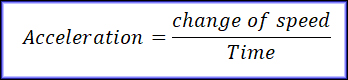Def. The rate of change of velocity of a body with respect to time is called its Acceleration.

Let,

Initial velocity of the body= u

Final velocity of the body= v

Time interval = t

Acceleration of the body = a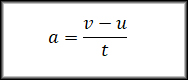Unit of Acceleration

MKS o SI method : metre / second2

CGS Method : centimeter / second2

FKS Method : foot / second2

### Problems:

A car is travelling at 54 km / h. If its velocity increases to 72 km / h in 5 second, then find the acceleration of the car in SI unit.

We know, metre / second2 are the unit of acceleration in SI unit.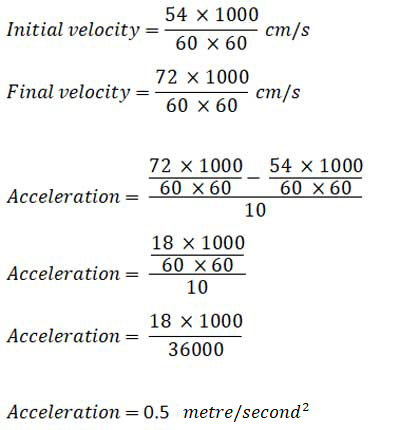### Retardation

If any rigid body with un-uniform speed decreases its velocity continuously then the rate of the decreasing speed is known as acceleration of the body. That is, decreasing of the velocity in respect of time is known as Retardation.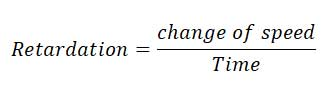Def. The rate of change of velocity of a body with respect to time is called its Retardation.

Let,

Initial velocity of the body= u

Final velocity of the body= v

Time interval = t

Retardation of the body = a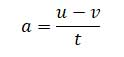### Unit of Retardation

MKS o SI method : metre / second2

CGS Method : centimeter / second2

FKS Method : foot / second2

### Problems:

A car is travelling at 72 km / h. If its velocity increases to 54 km / h in 5 second, then find the Retardation of the car in SI unit.

We know, metre / second2 are the unit of Retardation in SI unit.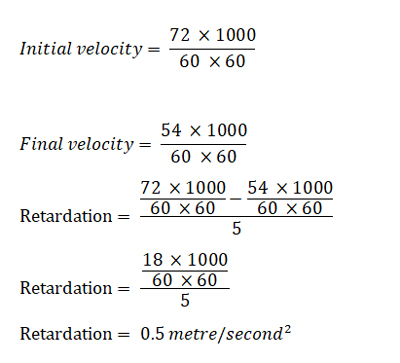Velocity Newtons law of motion - 1st Law
Author DetailsArnab De
I have over 16 years of experience working as an IT professional, ranging from teaching at my own institute to being a computer faculty at different leading institute across Kolkata. I also work as a web developer and designer, having worked for renowned companies and brand. Through tutorialathome, I wish to share my years of knowledge with the readers.
Enter New Comment
Comment History